# physics fun.

By Hal Canary, 2007-06-23 10:50:40 (link)
#physics

What is the tension in a spinning ring, for example Bank's orbitals or a Niven Ring?

Let us brake the ring into N sections. The angle (in radians) subtended by each section, dθ, is 2π / N. Suppose the radius of the ring is R and the mass-per-unit-length of the ring is λ; then the mass of a section is λRdθ and the mass of the whole ring M = 2πRλ.

Ignoring gravity, there are two forces pulling on each small section: tension pulling to the left and tension pulling to the right. Because the ring is circularly symmetric, the magnitude of the two tensions are equal. The directions of the two forces are dθ from being opposite.

If at some moment in time, the left tension is pointing in the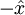$-\hat{x}$ direction, then the right tension will be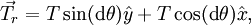$\vec{T}_{r} = T\sin({{\textrm{d}}\theta})\hat{y} + T\cos({\textrm{d}}\theta)\hat{x},$

where T is the magnatude of either tension.

As N gets large and dθ gets small,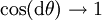$\cos({\textrm{d}}\theta)\rightarrow 1$ and$\sin({\textrm{d}}\theta)\rightarrow {\textrm{d}}\theta$. So: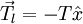$\vec{T}_{l} = -T\hat{x}$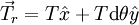$\vec{T}_{r} = T\hat{x} + T{\textrm{d}}\theta\hat{y}$

Consequently, the centripital force on the small section is Tdθ. From Newton's second law:

Tdθ = (λRdθ)a

where a is the centripetal acceleration.

T = λRa

Part B: Plug in some numbers.

Let P be the period of revolution. P = 2π√(R/a).

If the ring is made of solid steel, T / λ = 154000 N·m/kg. Setting our centripetal acceleration to an earthly 10 m/s², we find that the maximum radius for a ring of steel is 15 kilometers. Period of revolution = 243 seconds = 4 minutes. If you assume that half of the mass of your ring is structural material and the other half is nonstructural, then you get a diameter of 15 km. Multiply the period by 1/√2.

Carbon nanotubes: maximum size of 4800 kilometers. Period of revolution = 4353 seconds = 1.2 hours

The period of revolution for a Culture Orbital is a convenient 24 hours. The radius of a Niven Ring is 1 AU. Neither of these is feasible with today's understanding of physics.

On the other hand, a Stanford Torus with a radius of 893 meters and an orbital period of 60 seconds would need to have an average specific strength of 8745 N·m/kg, so at least 6% of the mass would need to be steel structure. (You would probably want a 4x fudge factor. Say 25% structural steel. Then sections of the ring could be replaced if they show signs of wear.)

(back)